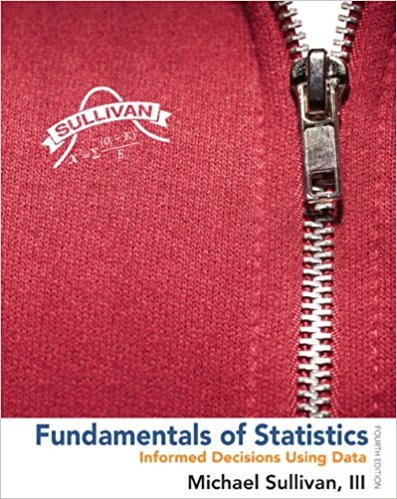×
×

# Solutions for Chapter Chapter 12.4: Fundamentals of Statistics 4th Edition## Full solutions for Fundamentals of Statistics | 4th Edition

ISBN: 9780321838704Solutions for Chapter Chapter 12.4

Solutions for Chapter Chapter 12.4
4 5 0 342 Reviews
21
0
##### ISBN: 9780321838704

Since 34 problems in chapter Chapter 12.4 have been answered, more than 305270 students have viewed full step-by-step solutions from this chapter. Fundamentals of Statistics was written by and is associated to the ISBN: 9780321838704. Chapter Chapter 12.4 includes 34 full step-by-step solutions. This expansive textbook survival guide covers the following chapters and their solutions. This textbook survival guide was created for the textbook: Fundamentals of Statistics, edition: 4.

Key Statistics Terms and definitions covered in this textbook
• Assignable cause

The portion of the variability in a set of observations that can be traced to speciic causes, such as operators, materials, or equipment. Also called a special cause.

• Asymptotic relative eficiency (ARE)

Used to compare hypothesis tests. The ARE of one test relative to another is the limiting ratio of the sample sizes necessary to obtain identical error probabilities for the two procedures.

• Binomial random variable

A discrete random variable that equals the number of successes in a ixed number of Bernoulli trials.

• Categorical data

Data consisting of counts or observations that can be classiied into categories. The categories may be descriptive.

• Conidence interval

If it is possible to write a probability statement of the form PL U ( ) ? ? ? ? = ?1 where L and U are functions of only the sample data and ? is a parameter, then the interval between L and U is called a conidence interval (or a 100 1( )% ? ? conidence interval). The interpretation is that a statement that the parameter ? lies in this interval will be true 100 1( )% ? ? of the times that such a statement is made

• Critical value(s)

The value of a statistic corresponding to a stated signiicance level as determined from the sampling distribution. For example, if PZ z PZ ( )( .) . ? =? = 0 025 . 1 96 0 025, then z0 025 . = 1 9. 6 is the critical value of z at the 0.025 level of signiicance. Crossed factors. Another name for factors that are arranged in a factorial experiment.

• Crossed factors

Another name for factors that are arranged in a factorial experiment.

• Cumulative normal distribution function

The cumulative distribution of the standard normal distribution, often denoted as ?( ) x and tabulated in Appendix Table II.

• Curvilinear regression

An expression sometimes used for nonlinear regression models or polynomial regression models.

• Defects-per-unit control chart

See U chart

• Degrees of freedom.

The number of independent comparisons that can be made among the elements of a sample. The term is analogous to the number of degrees of freedom for an object in a dynamic system, which is the number of independent coordinates required to determine the motion of the object.

• Designed experiment

An experiment in which the tests are planned in advance and the plans usually incorporate statistical models. See Experiment

• Discrete distribution

A probability distribution for a discrete random variable

• Error mean square

The error sum of squares divided by its number of degrees of freedom.

• Expected value

The expected value of a random variable X is its long-term average or mean value. In the continuous case, the expected value of X is E X xf x dx ( ) = ?? ( ) ? ? where f ( ) x is the density function of the random variable X.

• F-test

Any test of signiicance involving the F distribution. The most common F-tests are (1) testing hypotheses about the variances or standard deviations of two independent normal distributions, (2) testing hypotheses about treatment means or variance components in the analysis of variance, and (3) testing signiicance of regression or tests on subsets of parameters in a regression model.

• Factorial experiment

A type of experimental design in which every level of one factor is tested in combination with every level of another factor. In general, in a factorial experiment, all possible combinations of factor levels are tested.

• Fixed factor (or fixed effect).

In analysis of variance, a factor or effect is considered ixed if all the levels of interest for that factor are included in the experiment. Conclusions are then valid about this set of levels only, although when the factor is quantitative, it is customary to it a model to the data for interpolating between these levels.

• Fraction defective control chart

See P chart

• Goodness of fit

In general, the agreement of a set of observed values and a set of theoretical values that depend on some hypothesis. The term is often used in itting a theoretical distribution to a set of observations.

×